Next: Weak Oblique Shocks Up: Two-Dimensional Compressible Inviscid Flow Previous: Oblique Shocks

# Supersonic Flow in Corner or over Wedge

In practice, what determines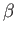and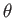? In other words, how are oblique shocks generated? In non-viscous flow, any streamline can be replaced by a solid boundary. Thus, the oblique shock flow, described in the previous section, provides the solution to the problem of supersonic flow in a concave corner, as illustrated in Figure 15.3. For given values ofand, the values ofand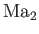are determined. [See Exercise i and Equation (15.7).] For the present, we shall only consider cases in which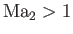. This restricts the oblique shock solution to the weaker of the two possible solutions. We also require thatbe less than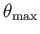. The stronger shock solution, as well as cases in which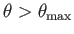, will be discussed later. (See Section 15.7.)

By symmetry, supersonic flow in a concave corner that turns though an angleis equivalent to one half of the flow pattern that results when a supersonic fluid is normally incident on a symmetric wedge of nose angle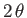. This is illustrated in Figure 15.4. It is clear, from the figure, that the presence of the wedge only affects the flow in the region that lies to the right of the two shock fronts that are attached to the wedge apex,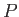. In particular, the presence of the apex atonly affects the flow in the region that lies to the right of the shock fronts.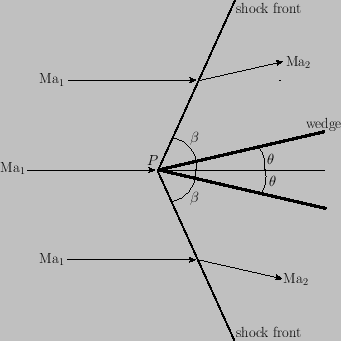The part of Figure 15.2 that we are presently considering (i.e.,) is such that a decrease in the wedge angle,, corresponds to a decrease in the wave angle,. Whendecreases to zero (causing the wedge to disappear),decreases to the limiting value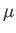. Moreover, the shock strength (which parameterizes the jump in quantities across the shock front) becomes zero. (See Section 15.4.) In fact, there is no disturbance in the flow in the limit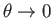. Thus, in Figure 15.4, there is no longer anything unique about the point; indeed, this point might correspond to any point in the flow. The angleis simply a characteristic angle associated with the local Mach number of the flow, according to the relation (15.6). As we have already mentioned,is known as the Mach angle. Note that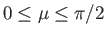for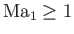.

The lines of inclination(with respect to the upstream directed streamline) that may be drawn at any point in the flow are known as Mach lines. At a general point,, there are always two lines that intersect the local streamline at the angle. (In three-dimensional flow, the Mach lines define a conical surface with apex, known as a Mach cone.) Thus, a two-dimensional supersonic flow pattern is associated with two families of Mach lines. These are conventionally distinguished by the labels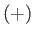and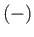. Those lying in theset run to the right of the (upstream directed) streamline, whereas those in theset run to the left. (See Figure 15.5.) Mach lines are also sometimes called characteristics. It is clear, from our previous discussion, that the flow conditions at pointcan only affect those at some other point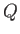if the latter point lies between theandMach lines passing through point. (See Figure 15.5, as well as Exercise ii.) Hence, we deduce that, unlike subsonic flow, there is no upstream influence in supersonic flow.

Oblique shocks can also be distinguished by the labelsand, according to which set of characteristics they asymptote to in the limit of zero shock strength.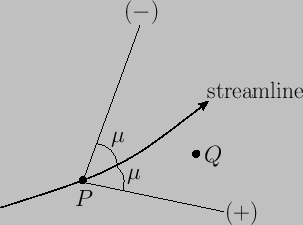Next: Weak Oblique Shocks Up: Two-Dimensional Compressible Inviscid Flow Previous: Oblique Shocks
Richard Fitzpatrick 2016-03-31